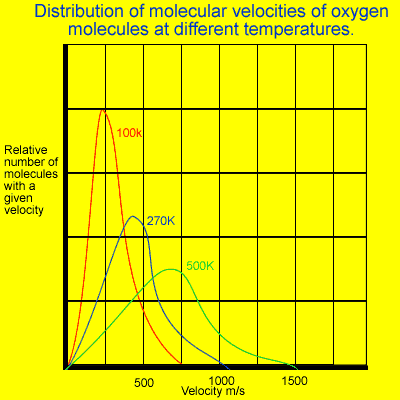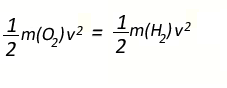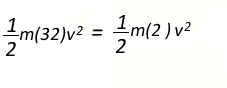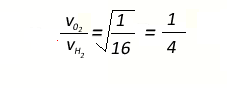Average kinetic energy of gas molecules Maxwell-Boltzmann distribution curveThe kinetic theory states that "The average kinetic energy of gas molecules is proportional to the absolute temperature of the gas". Not all molecules will have the same kinetic energy and hence the same speed. An increase in temperature increases the average speed of the molecules and the range of speeds. Look at the image on the left. It represents the Maxwell-Boltzmann distribution curve of kinetic energy of oxygen molecules at three different temperatures. The average kinetic energy of oxygen molecules and hydrogen molecules will be the same at any particular temperature. If we keep in mind that the formula for kinetic energy is 0.5mv2 it stands to reason that the lighter molecules(hydrogen) will have a greater speed than the oxygen molecules. Since the average kinetic energy of oxygen and hydrogen are equal we can write the expression below.However the molecular mass of oxygen is 32 and that of hydrogen is 2. So we can rewrite the above expressionTransforming the formula we obtain the expression below which gives the ratio of the average velocities of oxygen and hydrogen moleculesThe average velocity of hydrogen molecules is 4 times greater than that of oxygen molecules. It is important to note that although the average kinetic energy of all gases is the same at a particular temperature, the average velocity of the molecules is not. The heavier molecules tend to travel slower relative to the lighter ones. Three gases oxygen, nitrogen and hydrogen are placed in the same vessel at a certain temperature. If the average speed of the hydrogen molecules was measured at 500m/s calculate the average speed of the nitrogen and oxygen molecules. Clues Answer Continue with some exercises

Home